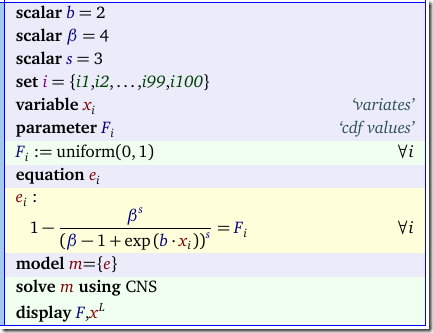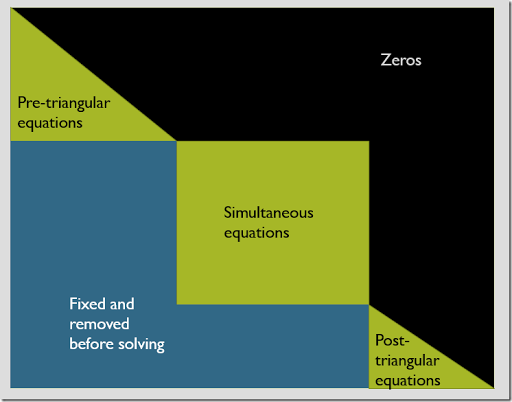## Saturday, May 6, 2017

### Nonlinear equations and CONOPT

##### The problem

When we want to generate some random draws from an exotic probability distribution, the following algorithm may help:

1. Generate $$u_i \sim U(0,1)$$ from a uniform distribution.
2. Solve $$F(x_i) = u_i$$ where $$F(x)$$ is the cumulative distribution function (cdf).

For most well-known distributions better methods exists, with better numerical properties especially in the tail areas. An example of such an less-known exotic distribution is the Gamma/Gompertz distribution (1).

##### Implementation

Here is a model I suggested to do the above:In the above model we form a system of nonlinear equations $$G(x)=F$$ in GAMS. It is a diagonal system: the Jacobian has entries on the diagonal only.

In most cases it is better to solve $$n$$ single equations instead of a system of $$n$$ equations. However there are a few reasons why this is not such a crazy approach as it looks:

• GAMS has a relatively high fixed cost overhead in setting up a model and calling a solver.
• A single model is a little bit more compact.
• I solved this model with CONOPT, and CONOPT will actually detect the diagonal structure and then solve the equations one by one. We can see this from some of the messages CONOPT produces.
##### Diagnostic messages

The log shows the following:

 C O N O P T 3   version 3.17C   Copyright (C)   ARKI Consulting and Development A/S                   Bagsvaerdvej 246 A                   DK-2880 Bagsvaerd, Denmark   Iter Phase Ninf   Infeasibility   RGmax    NSB   Step InItr MX OK     0   0        4.3170875683E+01 (Input point)                   Pre-triangular equations:   100                  Post-triangular equations:  0      1   0        0.0000000000E+00 (After pre-processing)     2   0        0.0000000000E+00 (After scaling)     2            0.0000000000E+00 ** Feasible solution to a square system.

When CONOPT receives the model it searches for triangular pieces of the model. The following graphic illustrates this:In this picture rows are equations and columns are variables. After reordering the rows and columns we have in left-upper corner a triangular part. Those equations we can solve one-by-one. In the middle we have the remaining simultaneous equations. This is the difficult part to solve. Finally we have a post-triangular part, which we can again solve one-by-one. Note that this presolve strategy is applied for optimization models as well (in that case the Jacobian matrix is usually not a square matrix).

For our “diagonal” example all equations fall in the pre-triangular part of the model. So indeed CONOPT does not solve this as a simultaneous equation problem.

##### Funny message

In the listing file we found one more message:

 It may be more efficient to turn the Post-triangular equationsinto ordinary equations with option: Lspost=False

Initially this reads a silly message: we have 0 post-triangular equations. However, it is the searching that is the expensive part. So even if there are eventually zero post-triangular equations, in some cases it is better to turn this search off. For our particular problem we don’t have to bother.

##### Results

The final results of this model look like:

 ----     21 PARAMETER F  cdf values i1   0.172,    i2   0.843,    i3   0.550,    i4   0.301,    i5   0.292,    i6   0.224,    i7   0.350,    i8   0.856i9   0.067,    i10  0.500,    i11  0.998,    i12  0.579,    i13  0.991,    i14  0.762,    i15  0.131,    i16  0.640i17  0.160,    i18  0.250,    i19  0.669,    i20  0.435,    i21  0.360,    i22  0.351,    i23  0.131,    i24  0.150i25  0.589,    i26  0.831,    i27  0.231,    i28  0.666,    i29  0.776,    i30  0.304,    i31  0.110,    i32  0.502i33  0.160,    i34  0.872,    i35  0.265,    i36  0.286,    i37  0.594,    i38  0.723,    i39  0.628,    i40  0.464i41  0.413,    i42  0.118,    i43  0.314,    i44  0.047,    i45  0.339,    i46  0.182,    i47  0.646,    i48  0.561i49  0.770,    i50  0.298,    i51  0.661,    i52  0.756,    i53  0.627,    i54  0.284,    i55  0.086,    i56  0.103i57  0.641,    i58  0.545,    i59  0.032,    i60  0.792,    i61  0.073,    i62  0.176,    i63  0.526,    i64  0.750i65  0.178,    i66  0.034,    i67  0.585,    i68  0.621,    i69  0.389,    i70  0.359,    i71  0.243,    i72  0.246i73  0.131,    i74  0.933,    i75  0.380,    i76  0.783,    i77  0.300,    i78  0.125,    i79  0.749,    i80  0.069i81  0.202,    i82  0.005,    i83  0.270,    i84  0.500,    i85  0.151,    i86  0.174,    i87  0.331,    i88  0.317i89  0.322,    i90  0.964,    i91  0.994,    i92  0.370,    i93  0.373,    i94  0.772,    i95  0.397,    i96  0.913i97  0.120,    i98  0.735,    i99  0.055,    i100 0.576 ----     21 VARIABLE x.L  variates i1   0.115,    i2   0.743,    i3   0.399,    i4   0.205,    i5   0.199,    i6   0.151,    i7   0.240,    i8   0.767i9   0.045,    i10  0.357,    i11  1.690,    i12  0.424,    i13  1.396,    i14  0.620,    i15  0.087,    i16  0.482i17  0.107,    i18  0.169,    i19  0.512,    i20  0.305,    i21  0.248,    i22  0.242,    i23  0.088,    i24  0.101i25  0.434,    i26  0.721,    i27  0.156,    i28  0.508,    i29  0.638,    i30  0.207,    i31  0.074,    i32  0.358i33  0.107,    i34  0.799,    i35  0.180,    i36  0.194,    i37  0.438,    i38  0.571,    i39  0.471,    i40  0.327i41  0.288,    i42  0.079,    i43  0.215,    i44  0.031,    i45  0.232,    i46  0.122,    i47  0.488,    i48  0.408i49  0.630,    i50  0.203,    i51  0.503,    i52  0.612,    i53  0.470,    i54  0.193,    i55  0.058,    i56  0.069i57  0.483,    i58  0.395,    i59  0.021,    i60  0.662,    i61  0.049,    i62  0.118,    i63  0.378,    i64  0.605i65  0.120,    i66  0.023,    i67  0.430,    i68  0.464,    i69  0.270,    i70  0.247,    i71  0.164,    i72  0.167i73  0.087,    i74  0.964,    i75  0.263,    i76  0.649,    i77  0.204,    i78  0.084,    i79  0.603,    i80  0.046i81  0.136,    i82  0.003,    i83  0.183,    i84  0.356,    i85  0.101,    i86  0.117,    i87  0.226,    i88  0.216i89  0.220,    i90  1.105,    i91  1.460,    i92  0.255,    i93  0.257,    i94  0.633,    i95  0.275,    i96  0.898i97  0.080,    i98  0.586,    i99  0.037,    i100 0.422
##### Notes

As noted in the comments this particular CDF can be solved directly using:

 $x_i = \frac{\ln\left(\beta (1-F_i)^{-1/s}-\beta+1 \right)}{b}$
##### References
1. Gamma/Gompertz distribution, https://en.wikipedia.org/wiki/Gamma/Gompertz_distribution